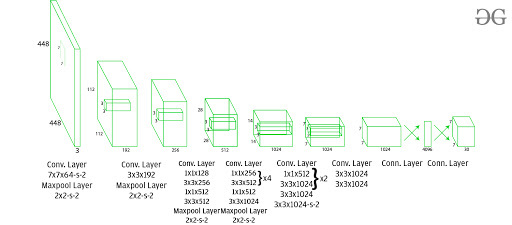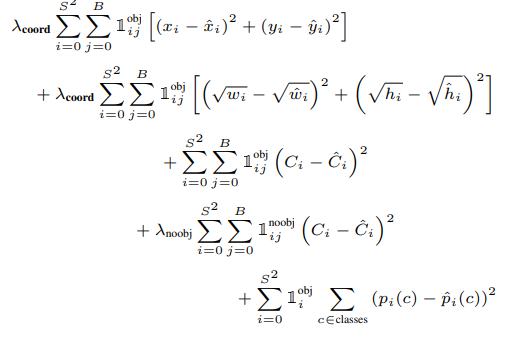# YOLO : You Only Look Once – Real Time Object Detection

YOLO was proposed by Joseph Redmond et al. in 2015. It was proposed to deal with the problems faced by the object recognition models at that time, Fast R-CNN is one of the state-of-the-art models at that time but it has its own challenges such as this network cannot be used in real-time, because it takes 2-3 seconds to predicts an image and therefore cannot be used in real-time.

Architecture:This architecture takes an image as input and resizes it to 448*448 by keeping the aspect ratio same and performing padding. This image is then passed in the CNN network. This model has 24 convolution layers, 4 max-pooling layers followed by 2 fully connected layers. For the reduction of the number of layers (Channels), we use 1*1 convolution that is followed by 3*3 convolution. Notice that the last layer of YOLOv1 predicts a cuboidal output. This is done by generating (1, 1470) from final fully connected layer and reshaping it to size (7, 7, 30).

This architecture uses Leaky ReLU as its activation function in whole architecture except layer where it uses linear activation function. The definition of Leaky ReLU can be found here.Batch normalization also helps regularize the model. With batch normalization, we can remove dropout from the model without overfitting it.

Training:
This model is trained on the ImageNet-1000 dataset. The model is trained over a week and achieve top-5 accuracy of 88% on ImageNet 2012 validation which is comparable to GoogLeNet (2014 ILSVRC winner), the state of the art model at that time. Fast YOLO uses fewer layers (9 instead of 24) and fewer filters. Except this, the fast YOLO have all parameters similar to YOLO.YOLO uses sum-squared error loss function which is easy to optimize. However, this function gives equal weight to the classification and localization task. The loss function defined in YOLO as follows:where,denotes if object is present in cell i.denotesbounding box resposible for prediciton of object in the cell i.andare regularisation parameter required to balance the loss function.
In this model, we takeandThe first two parts of the above loss equation represent localization mean-squared error, but the other three parts represent classification error. In the localization error, the first term calculates the deviation from the ground truth bounding box. The second term calculates the square root of the difference between height and width of the bounding box. In the second term, we take the square root of width and height because our loss function should be able to consider the deviation in terms of the size of the bounding box. For small bounding boxes, the little deviation should be more important as compared to large bounding boxes.
There are three terms in classification loss, the first term calculates the sum-squared error between the predicted confidence score and the ground truth for each bounding box in each cell. Similarly, The second term calculates the mean-squared sum of cells that do not contain any bounding box, and a regularization parameter is used to make this loss small. The third term calculates the sum-squared error of the classes belongs to these grid cells.

Detection:
This architecture divides the image into a grid of S*S size. If the centre of the bounding box of the object is in that grid, then this grid is responsible for detecting that object. Each grid predicts bounding boxes with their confidence score. Each confidence score shows how accurate it is that the bounding that predicts contains an object and how precise it predicts the bounding box coordinates wrt. ground truth prediction.

At test time we multiply the conditional class probabilities and the individual box confidence predictions. We define our confidence score as follows :Note, the confidence score should be 0 when there is no object exists in the grid. If there is an object present in the image the confidence score should be equal to IoU between ground truth and predicted boxes. Each bounding box consists of 5 predictions: (x, y, w, h) and confidence score. The (x, y) coordinates represent the centre of the box relative to the bounds of the grid cell. The h, w coordinates represents height, width of bounding box relative to (x, y). The confidence score represents the presence of an object in the bounding box.

This results into combinatation of bounding boxes from each grid like this.

Each grid also predict C conditional class probability, Pr (Classi | Object).

This probability were conditional based on the presence of an object in grid cell. Regardless the number of boxes each grid cell predicts only one set of class probabilities. Thes prediction are encoded in the 3D tensor of size S * S * (5*B +C).

Now,we multiply the conditional class probabilities and the individual box confidence predictions,which gives us class-specific confidence scores for each box. These scores encode both the probability of that class appearing in the box and how well the predicted box fits the object. Then after we apply non-maximal suppression to predict generate the final result of the input
YOLO is very fast at the test time because it uses only a single CNN architecture to predict results and lass is defined in such a way that it treats classification as a regression problem.

Results:
The simple YOLO has mAP of 63.4% when trained on VOC 2007 and 2012, the Fast YOLO which is almost 3x faster in result generation has mAP of 52%. This is lower than the best Fast R-CNN model achieved (71% mAP) and also the R-CNN achieved (66% mAP). However, it beats other real-time detectors such as (DPMv5 33% mAP) on accuracy.

Benefits of YOLO:

• Process frames at the rate of 45 fps (larger network) to 150 fps(smaller network) which is better than real-time.
• The network is able to generalize the image better.

• Comparatively low recall and more localization error compared to Faster R_CNN.
• Struggles to detect close objects because each grid can propose only 2 bounding boxes.
• Struggles to detect small objects.

My Personal Notes arrow_drop_upCheck out this Author's contributed articles.

If you like GeeksforGeeks and would like to contribute, you can also write an article using contribute.geeksforgeeks.org or mail your article to contribute@geeksforgeeks.org. See your article appearing on the GeeksforGeeks main page and help other Geeks.

Please Improve this article if you find anything incorrect by clicking on the "Improve Article" button below.

Article Tags :
Practice Tags :

Be the First to upvote.

Please write to us at contribute@geeksforgeeks.org to report any issue with the above content.